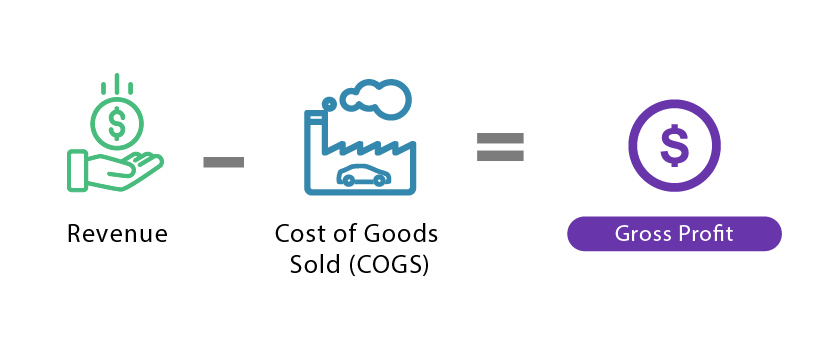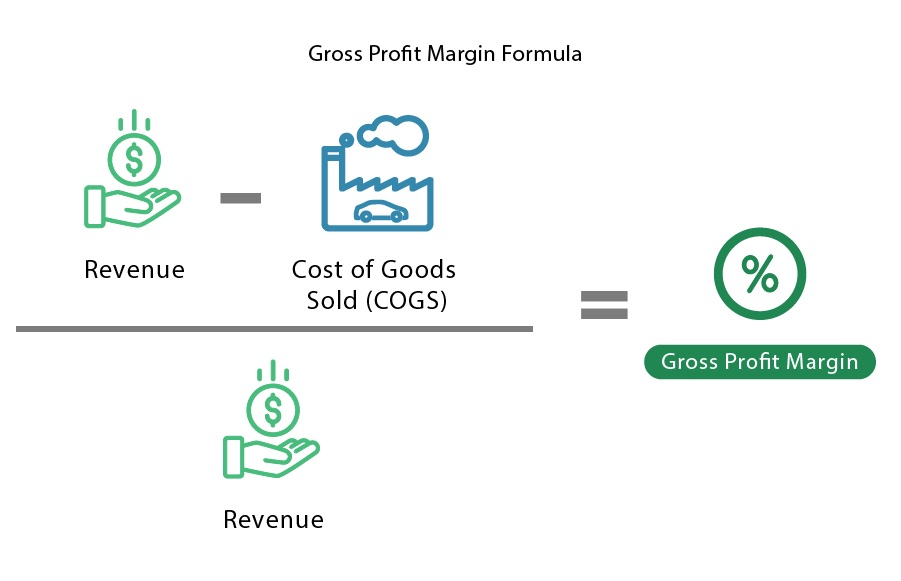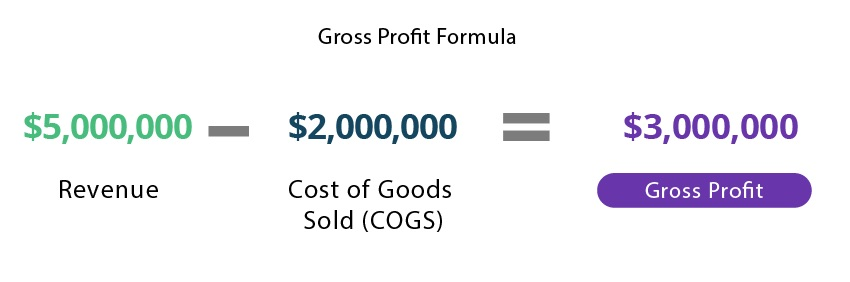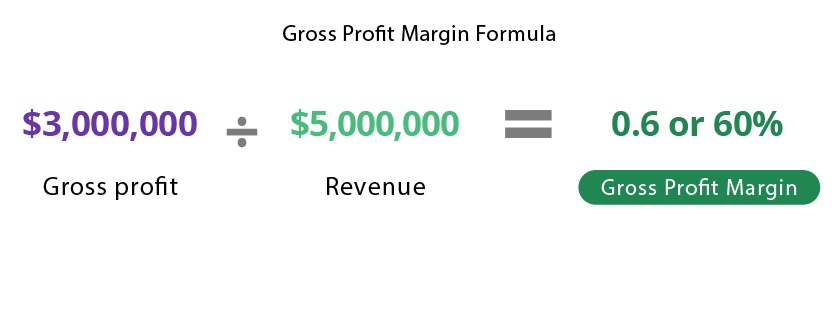# Gross Profit Margin

Reviewed by:
ImageWritten by:
ImageUpdated August 24, 2020

## What Is Gross Profit Margin?

Gross profit margin is a measure of a company’s profitability, calculated as the gross profit as a percentage of revenue. Gross profit is the amount remaining after deducting the cost of goods sold (COGS) or direct costs of earning revenue from revenue.

Note that cost of goods sold is a measure of the direct costs required to produce a good or service (like materials and labor). It excludes indirect expenses like distribution costs, marketing, and accounting. This makes the gross profit margin only useful for tracking the direct cost of operations as a percentage of sales. Other profit ratios, such as net profit margin, reflect different measures of profit.

## How to Calculate Gross Profit Margin

Gross profit is calculated by subtracting cost of goods sold from revenue:### Gross Profit Margin Formula

Gross profit margin (which is a percentage) is calculated by dividing gross profit by revenue:## Gross Profit Margin Example

Say a company earned \$5,000,000 in revenue by selling shoes, and the shoes created \$2,000,000  of labor and materials costs to produce. To calculate gross profit, the company subtracts cost of goods sold from revenue:To get gross profit margin, divide gross profit by revenue:This means that the direct costs of producing the product that the company sells consume 40% of its revenue, and that it has 60% of its revenue to cover indirect costs and create profit for the owners.

## Should Gross Profit Margin Be High or Low?

Gross profit margin should be high, as a higher margin means that there is more available to invest, save, and/or cover indirect expenses. A higher gross profit margin indicates that a company is making more profit on sales and is therefore more efficient at converting raw materials into income. A lower profit margin usually means that a company is less efficient at converting raw materials into income and therefore is making less profit from its sales.

Many investors and analysts use gross profit margin to gauge a company’s profitability in order to compare it with competitors. The percentage is also used to track a company’s progress over time. Since a higher margin can indicate increased efficiency and greater earning potential, investors may be willing to pay more for companies with higher profit margins.

## What Is a Good Gross Profit Margin?

The definition of a “good” gross profit margin varies by industry, but generally speaking, 5% is low, 10% is average, and 20% is considered a “good” gross profit margin. However, here is an overview of average gross profit margins across various industries.

## What Does a Low Gross Profit Percentage Mean?

A lower (or decreasing) gross profit margin indicates that a company is creating less gross profit from its revenue and is therefore less efficient at turning raw materials and labor into income. This means that it has less money to put towards savings, operations, and/or indirect expenses. It may indicate that there are problems within the company, such as overpriced production inputs or underpriced products.

Every financial ratio has its pros and cons, and while there are a number of reasons why gross profit margin can be useful, there are also some potential disadvantages:

### Advantages of Gross Profit Margin

• Straightforward. This simple calculation provides a wealth of information: It indicates cost efficiency, helps companies track performance over time, etc.

• Pricing control. Using profit margin as a guideline, companies can adjust their pricing strategies to optimize operations.

• Benchmarks. This measure allows companies to compare performance to industry averages and competitors.

• Potential improvements. By highlighting the effectiveness of individual products or services, gross profit margin can help companies identify areas for potential improvement.

### Disadvantages of Gross Profit Margin

• Doesn’t include all costs. This metric doesn’t account for costs such as taxes, marketing, accounting, and more (which limits its applications).

• Industry variance. Costs, profits, and margins vary greatly between industries, which could make it tough to set benchmarks or compare to other companies.

• Potentially misleading. Profit margins measure profitability but they don’t always reflect underlying activities. For example, some companies reduce their prices to increase their market share or spend more on production costs to secure a supplier.

• Should be combined with other metrics. Since gross profit margin only considers production costs, it’s not always the most accurate indicator of profitability. To get around this, companies and analysts should use it in combination with other metrics.

## Gross Profit vs. Gross Profit Margin

Gross profit and gross profit margin both gauge the profitability of a company by measuring revenue with respect to costs of production. However, the main difference is that gross profit is a value, whereas gross profit margin is a percentage.

Gross profit indicates how much revenue a company has after deducting the costs of production. It is calculated by subtracting the cost of goods sold from revenue, which shows the amount that can finance indirect expenses and investments.

Gross profit margin indicates the gross profit as a percentage of revenue and is calculated by dividing gross profit by revenue. This percentage value indicates the proportion of revenue that is not consumed by the direct costs of producing the goods or services for sale. It indicates how efficiently the company generates gross profit from revenue. The higher the margin, the more a company earns for each dollar spent on production.

## Gross Profit Margin vs. Net Profit Margin

Gross profit margin and net profit margin are two measures that are both used to calculate the profitability of a company, but there is one key difference:

Gross profit margin is a measure of the proportion of  revenue left after accounting for production costs. It illustrates how much profit a company earns in relation to each dollar spent on production. It is calculated by dividing gross profit (revenue - COGS) by revenue.

Net profit margin, on the other hand, is a measure of the proportion of  revenue left after ALL expenses are accounted for. This metric indicates how each dollar of revenue translates into profit. It is calculated by dividing net profit (gross profit - operating expenses and all other expenses) by revenue.